ts_new

### Introduction¶

A recent talk by Vincent Warmerdam about how simple models can outperform state-of-the-art ML approaches was a real eye opener to me. What amazed me the most while watching the video, is his astonishing creativity when it comes to feature creation and engineering. Although it is commonly known that good features are far more important than using the latest 10-pages-of-math Neural Network variation from arxiv, I regularly find myself guilty of trying to use the fanciest model available. In this post I took a step back and focused on a more creative idea to build a good and reliable time-series model. Besides the video mentioned before, a recent paper by Mark J. van der Laan et al. about an estimator called "Highly adaptive Lasso"(HAL) provided major inspiration for this idea.

In :
import pandas as pd
import numpy as np

from sklearn.linear_model import *

from copy import deepcopy

import matplotlib.pyplot as plt

import multiprocessing as mp

import warnings
warnings.filterwarnings('ignore')


### The dataset¶

Besides simplicity, I was also aiming for a model with high forecasting performance. The first dataset that I was testing, is the commonly used shampoo-sales dataset that - surprise - captures the cumulated monthly sales of some shampoo brand.

In :
df = pd.read_csv("shampoo.csv", index_col = 0)

fig, ax = plt.subplots(figsize = (12,6))
ax.plot(df)
fig.autofmt_xdate()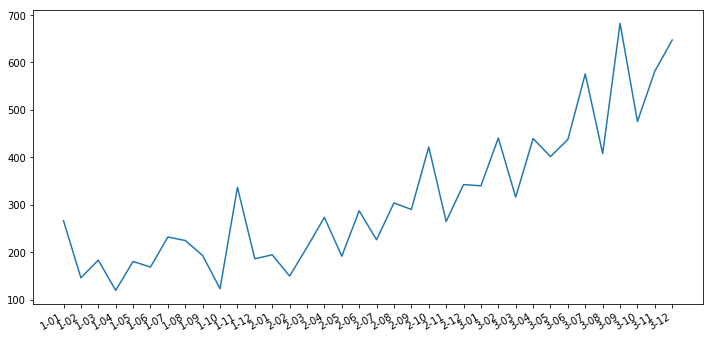The data looks rather unspectacular, probably a non-linear trend and some irregular spikes that might indicate an autoregressive/moving average component.

### The model¶

As the title suggests, the main feature of this idea is the indicator function. Closely related to a harmonic regression, this model will use periodically repeating variables as exogenous regressors. More specifically, a couple of indicator functions is being generated that take on a value of "1" in a periodic manner and "0" else. Formally, we define the starting time of the time-series as $t=0$ and specify another variable $\phi$ that specifies the frequency of the 0-1 pattern. An arbitrary regressor $x_{1;t}$ then takes on values

$$x_{1;t} = \mathbb{I}_{0}(t\,mod\,\phi)$$

with $mod$ being the modulo operator, $\mathbb{I}_{0}(\cdot)$ an indicator function that is "1" whenever its argument is zero and "0" else.

Let's define the indicator function and another function that allows vector-valued application of that indicator in Python:

In :
def period_indicator(t, phi):

if t%phi != 0:
return(0)

elif t%phi == 0:
return(1)

def period_indicator_vec(t, phi):

t_vec = pd.Series(t)

return(t_vec.apply(lambda x: period_indicator(x,phi)))


For a monthly time series over three years and a period of 12 that starts in the first month, a feature $x_1;t,\, t\in[0,35],\,\phi=12$ would look like this:

In :
plt.plot(period_indicator_vec(np.arange(36),12))

plt.xlabel("Time")

Out:
Text(0.5,0,'Time')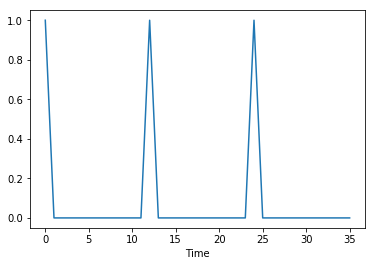(Although matplotlib interpolates to continuous time, the feature should actually only be equal to one 1 at $t=0,\,t=11,\,t=23$)

The first question that comes to mind: "What if the periodic pattern doesn't start in the first month?"
Although slightly clumsy, creating 11 more variables, each with different starting points for the periodic patterns turned out to be very effective (plotting all 12 possibilities looks kinda ugly, so I only plotted the first three variables - you should get the idea):

In :
for i in range(3):
plt.plot(period_indicator_vec(np.arange(i,36+i),12))
plt.xlabel("Time")

Out:
Text(0.5,0,'Time')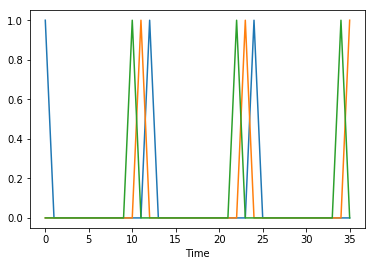The underlying assumption of this example is a yearly recurring effect that only occurs at the exact point in discrete time and nowhere else. This idea can be developed further for example to quarterly occuring effects $\phi=3$ or whatever. Notice that for each pattern we also need $\phi$ variables with different starting points to capture all possibilities. Since this means a very quick increase in variables, I decided to restrict the maximum $\phi$ to a reasonable value for the problem at hand. In the case of the shampoo data, allowing a maximal $\phi=12$ to express a yearly recurring pattern at maximum, was reasonable.

### Applying the model to the data¶

The 'get_features()' function below will generate the periodic indicators according to the rules mentioned in 2). Since the shampoo time-series looks like it exhibits a quadratic trend, I decided to add an additive trend and an additive quadratic trend variable as well:

In :
def get_features(time_series, max_phi=12):

ts = np.array(time_series)

T = len(ts)

harmonics_list = []

for phi in range(1,np.minimum(T,max_phi)+1):
for i in range(phi):
indicator = period_indicator_vec(np.arange(i,T+i), phi)

harmonics_list.append(indicator)

harmonics_frame = pd.concat(harmonics_list,1)

harmonics_frame["trend"] = np.arange(T)

harmonics_frame["trend_sq"] = np.arange(T)**2

return harmonics_frame

In :
ts_features = get_features(df)


After the features have been created it is time to think of an actual algorithm to perform actual forecasts. In the manner of HAL estimator and considering that a sparse result is easier to interpret, the algorithm of choice was simple Lasso. To find a good value for Lasso's alpha parameter, the actual training set is separated in a train-validate split fashion and then grid-search is performed to select the best alpha based on the performance on the validate-set:

In :
#an extra class for the Lasso made it easier to deal with the pandas Series type - but that could probably
#be left out
class LassoForecaster:

def __init__(self):
pass

def fit(self, X,y, alpha = 1.,max_iter = 1000):
X_array = np.array(X)
y_array = np.array(y).reshape(-1,1)

self.model = Lasso(alpha = alpha, max_iter = max_iter)
self.model.fit(X_array,y_array)

def predict(self, X):
X_array = np.array(X)

predictions = self.model.predict(X_array).reshape(-1,1)

return predictions

#I hated to wait for unneccesarily long for the grid-search results, so I parallelized it
#This function will be used in a multiprocessing.Pool()

def alpha_grid_search(inp):

alpha = inp["alpha"]
X = np.array(inp["X"])
y = np.array(inp["y"]).reshape(-1,1)

train_size = 0.75
split_point = int(len(X)*train_size)

X_train = X[:split_point,:]
X_test = X[split_point:,:]

y_train = y[:split_point,:].reshape(-1,1)
y_test = y[split_point:,:].reshape(-1,1)

model = Lasso(alpha = alpha)
model.fit(X_train,y_train)

predictions = model.predict(X_test)

residuals = y_test - predictions

rmse = np.sqrt(np.mean(residuals**2))

return rmse


Now, the whole data is split into a training and a test set. The alpha grid search is performed on the training set only, while the actual performance is measured on the training set; I decided to forecast one year ahead (=12 months). Since the model doesn't need any lagged input variables, a simple train-test split like the one below corresponds to a true 12 steps ahead test (and not just one step ahead, as is often done).

In :
oos_size = 12

In :
X_train = ts_features.iloc[:-oos_size,:]
X_test = ts_features.iloc[-oos_size:,:]

y_train = df.iloc[:-oos_size]
y_test = df.iloc[-oos_size:]


At next, the grid search is performed to find an optimal value for alpha:

In :
proposed_alpha = np.arange(.002,1.,.002)

parallel_list = [{"alpha":alpha, "X": X_train, "y": y_train} for alpha in proposed_alpha]

pool = mp.Pool(processes = 16)
results = [pool.apply(alpha_grid_search,args=(inp,)) for inp in parallel_list]

In :
optimal_alpha = proposed_alpha[np.argmin(results)]


Final model fit, in-sample prediction and out-of-sample forecast:

In :
m = LassoForecaster()
m.fit(X_train,y_train,alpha = optimal_alpha, max_iter = 10000)

plt.figure(figsize = (12,6))
plt.plot(y_train.values)
plt.plot(m.predict(X_train))

plt.plot(len(y_train)-1+np.arange(len(y_test)),y_test.values)
plt.plot(len(y_train)-1+np.arange(len(y_test)),m.predict(X_test))
plt.xlabel("Time")
plt.legend(["In-sample truth", "In-sample prediction", "Out-of-sample truth", "Out-of-sample forecast"])

Out:
<matplotlib.legend.Legend at 0x7feb53c8d748>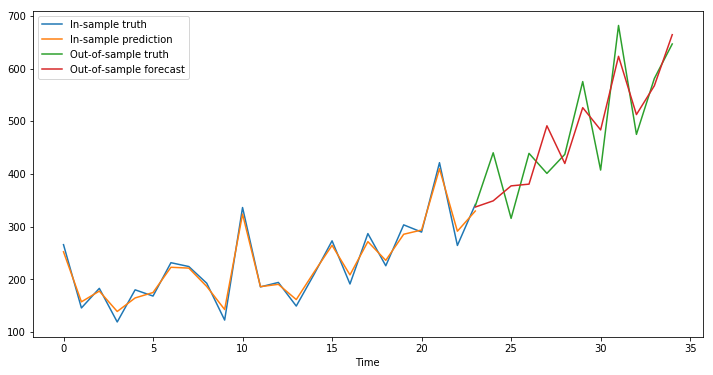Now comes the moment of truth with the out-of-sample forecasting error (I chose RMSE):

In :
print("RMSE:")
print(np.sqrt(np.mean((y_test.values-m.predict(X_test))**2)))

RMSE:
55.973300349921345


I found some blogs that used much more complex Machine Learning models (e.g. LSTMs) to forecast the same data over the same period and their RMSE was oftentimes much higher (RMSE > 100 in most cases). If you don't trust me here, feel free to google some examples - By no means do I want to diminish the effort of other people or appear superior but I think this is a good example that Machine Learning doesn't always mean better results.

### Takeaway¶

Thanks to Warmerdam's talk, I was really intrigued to take a step back from the Machine Learning intense approach towards forecasting that I am currently taking in my thesis (e.g. Bayesian Neural Networks) and try make a simple and interpretable model work. By extracting the non-zero factors from the Lasso model and the corresponding variable values, it would even be possible to fully interpret the model and decompose the output which is always a good way to sanity check (again, watch Warmderdam's talk!)

Although Google's AutoML could really revolutionize the whole Machine Learning community for the x-th time, I believe that it won't easily work with time-series like the one above. The main issue that I see here is the small amount of data available that - in my opinion - requires more domain expertise than more computational power to yield highly accurate forecasts. There are probably countless more tricks that can be applied to make simple linear models more powerful than they are currently being perceived by many people. I will happily present more ideas for that in the future.

### Bonus - Dealing with increasing amplitudes¶

Another dataset that is also in high usage for testing time-series models, is the airline passengers dataset.

In :
df = pd.read_csv("passengers.csv", index_col = 0)

plt.figure(figsize = (12,6))
plt.plot(df)
fig.autofmt_xdate()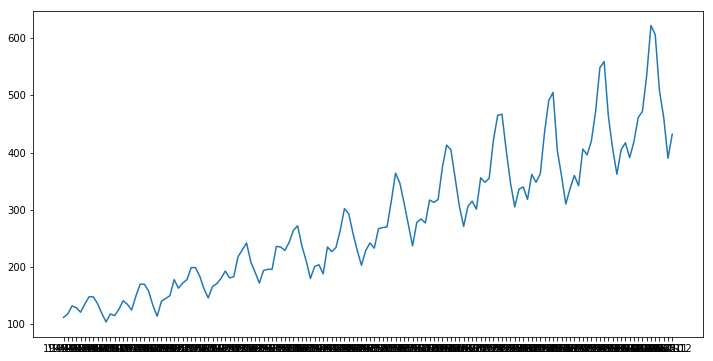By looking at the plot, the increasing amplitude of the realizations pierces the eye. Using the approach from above, the model output would result in a somewhat "mean"-amplitude over all periods, clearly not capturing the increase. To allow the model to incorporate this effect as well, each periodic indicator variable is multiplied with the square-root of the trend variable and saved as another feature:

$$\tilde{x_{1;t}} = \sqrt{t}\cdot\mathbb{I}_{0}(t\,mod\,\phi)$$

which looks like the removal of heteroscedasticity in classic linear models from econometrics. On the other hand, the quadratic trend variable is removed and only the simple linear trend variable is kept.

In :
def get_features_het(time_series, max_phi=12):

ts = np.array(time_series)

T = len(ts)

harmonics_list = []

for phi in range(1,np.minimum(T,max_phi)+1):
for i in range(phi):
indicator = period_indicator_vec(np.arange(i,T+i), phi)

harmonics_list.append(indicator)

hetskad = indicator * (np.arange(T)+1)**(1/2)

harmonics_list.append(pd.Series(hetskad))

harmonics_frame = pd.concat(harmonics_list,1)

harmonics_frame["trend"] = np.arange(T)

return harmonics_frame

In :
ts_features = get_features_het(df)


Since there is much more data available now, the out-of-sample size is increased to 3 years - everything else stays the same

In :
oos_size = 36

In :
X_train = ts_features.iloc[:-oos_size,:]
X_test = ts_features.iloc[-oos_size:,:]

y_train = df.iloc[:-oos_size]
y_test = df.iloc[-oos_size:]

In :
proposed_alpha = np.arange(.002,1.,.002)

parallel_list = [{"alpha":alpha, "X": X_train, "y": y_train} for alpha in proposed_alpha]

pool = mp.Pool(processes = 16)
results = [pool.apply(alpha_grid_search,args=(inp,)) for inp in parallel_list]

In :
optimal_alpha = proposed_alpha[np.argmin(results)]

In :
m = LassoForecaster()
m.fit(X_train,y_train,alpha = optimal_alpha, max_iter = 10000)

plt.figure(figsize = (12,6))
plt.plot(y_train.values)
plt.plot(m.predict(X_train))

plt.plot(len(y_train)-1+np.arange(len(y_test)),y_test.values)
plt.plot(len(y_train)-1+np.arange(len(y_test)),m.predict(X_test))
plt.xlabel("Time")
plt.legend(["In-sample truth", "In-sample prediction", "Out-of-sample truth", "Out-of-sample forecast"])

Out:
<matplotlib.legend.Legend at 0x7feb53279320>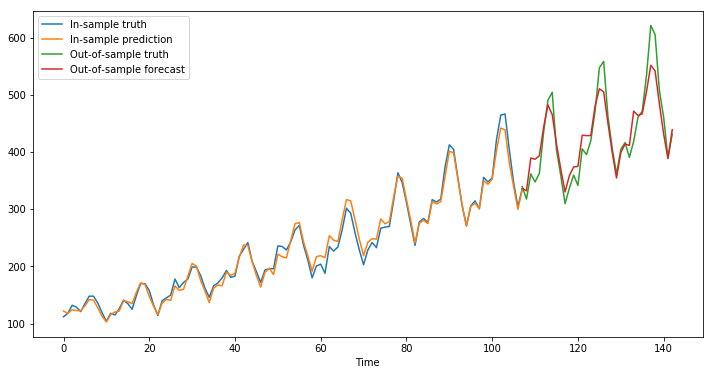In :
print("RMSE:")
print(np.sqrt(np.mean((y_test.values-m.predict(X_test))**2)))

RMSE:
28.071196336221476


Note: This is also below the error of many ML models that you can find after some google :)

### 6 Comments

1.#### Julius

Danke Sarem, super cooler Blog Post und super Ideen! Das hat wirklich Spaß gemacht 🙂

•#### Sarem

Danke Julius 😉

2.#### Marcus

Hey Sarem, really nice blog post. I’m attempting to implement this in a pet project myself. just out of curiosity, if you knew that the time series were affected by seasonal features such as public holidays, how would you go about adding these regressors in?

•#### Sarem

Hey Marcus,

thanks for your message. If the seasonal feature isn’t perfectly correlated with the indicator-features, you can just put them in as another feature. As an example, Sundays would be fully collinear with a period-7 indicator function and hence you should not add it as another feature unless you drop the period-7 indicator – the only reason that I can think of for that would be an easier interpretation of a ‘Sunday’ than some ‘period 7_X’ feature-name. A public holiday like 4th of July on the other hand would not be collinear with any of the base indicator-features and you would want to use that in you model as well.

Let me know if you need anything else.

Best,
Sarem

•#### Marcus

Thanks for responding! And again, really enjoy this post. I hope you’ll keep em coming.

•#### Sarem

I’ll try my best. Good luck on your project!